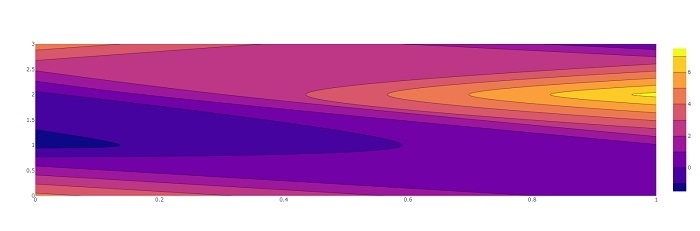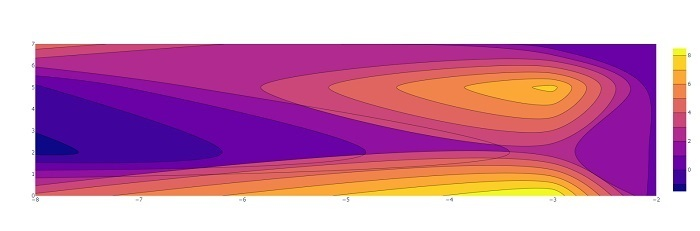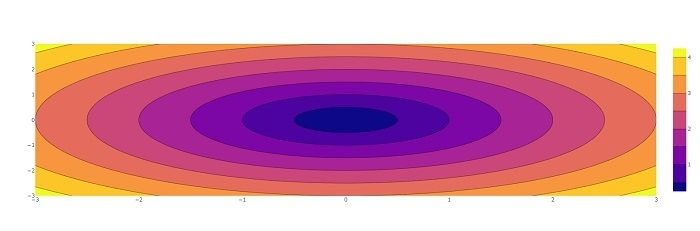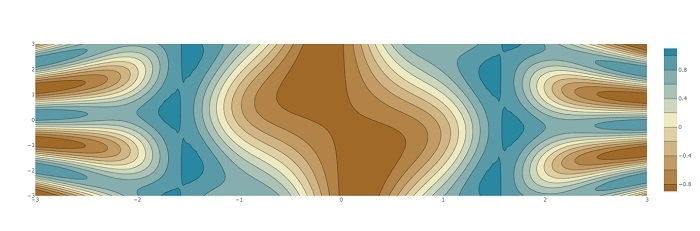# Contour Plots using Plotly in Python

In python Plotly referred as "plotly.py". It is a free and open-source plotting library that is built on top of “plotly.js”. It supports more than 40 unique chart types. This library is mainly used for financial, geographical, scientific, 3-dimensional, and data analysis applications.

It can be used to plot various types of charts and graphs like scatter plots, line plots, bar charts, box plots, histograms, pie charts, area charts, box plots, histograms, heatmaps, subplots, multiple-axes, etc.

## Installing plotly

Execute the below commands in the command prompt to install the plotly module. It is an easy way to install the latest package of Plotly from PyPi.

pip install plotly


A Contour plot is used for visualizing three-dimensional data in a two-dimensional surface by plotting constant z slices, called contours.

It is drawn with the help of a contour function (Z), which is a function of two inputs X, and Y (the X-axis and Y-axis coordinates).

Z = fun(x,y)


The ploty module provides a function called Contour, which is used to plot the contour plots.

## The contour() function

The plotly.graph_objects provides a method contour() to draw contour plots. The function has more than 50 parameters, here we will discuss a few parameters only.

### Syntax

plotly.graph_objects.Contour(z=None,x=None,y=None,arg=None,colorbar=None,hoverinfo=None,x=None,y=Non,**kwargs)


### Parameters

• z: By default None, two-dimensional list of values to compute the contour lines (z data).

• x: x coordinates, by default set to None.

• y: y coordinates, by default set to None.

## Contour Plot with a 2-D array as z-function

Plot the contour plot using a two dimentional array as a z-function.

### Example

In this example, we will plot a simple contour plot using the 2-dimenioanl array.

import plotly.graph_objects as go
fig = go.Figure(data = go.Contour(z=[[4.3, 0.2],
[-1.3, 0.9],
[-0.32, 7.3],
[4.6, 0.203]]))
fig.show()


### OutputHere the 4X2 array represents the z function.

## Contour plot with X and Y coordinates

Plot the contour plot using X and Y coordinates along with the z-function (a two dimensional array).

### Example

In this example, we will plot a contour plot using the 2-dimenioanl array along with X and Y coordinates.

import plotly.graph_objects as go

fig = go.Figure(data = go.Contour(z=[[4.3, 9, 0.2],
[-1.3, 2.3, 0.9],
[-0.32, 7.3, 0.23],
[4.6, 0.203, 0.34]],
x=[-8, -3, -2,-1, 0.23], # horizontal axis
y=[0, 2, 5, 7, 3]# vertical axis
))
fig.show()


### OutputHere x and y coordinates represents the horizontal and vertical axis respectively.

## Contour Plot using Numpy

Plot the contour plot using numpy, here we will use the numpy.meshgrid() function to produce arrays for X and Y coordinates.

### Example

The z-function will be sum of square root of x and y value using the numpy.sqrt() function.

import numpy as np
import plotly.graph_objects as go

xlist = np.linspace(-3.0, 3.0, 100)
ylist = np.linspace(-3.0, 3.0, 100)

# create a mesh
X, Y = np.meshgrid(xlist, ylist)
Z = np.sqrt(X**2 + Y**2)

trace = go.Contour(x = xlist, y = ylist, z = Z)
data = [trace]
fig = go.Figure(data)
fig.show()


### Output## Contour plot with colorscale

The colorscale is a parameter of the plotly.graph_objects.Contour() function which is used to set the colorscale.

### Example

Let’s take an example, and set the palette name string 'Earth' to the colorscale parameter.

import plotly.graph_objects as go
import numpy as np

def f(x, y):
return np.sin(x) ** 10 + np.cos(10 + y * x) * np.cos(x)

xlist = np.linspace(-3.0, 3.0, 100)
ylist = np.linspace(-3.0, 3.0, 100)

# A mesh is created with the given co-ordinates by this numpy function
X, Y = np.meshgrid(xlist, ylist)
Z = f(X,Y)

fig = go.Figure(go.Contour(x = xlist, y = ylist, z = Z, colorscale='Earth'))
fig.show()


### OutputWe have drawn the contour plots using the different z functions.

Updated on: 30-May-2023

154 Views